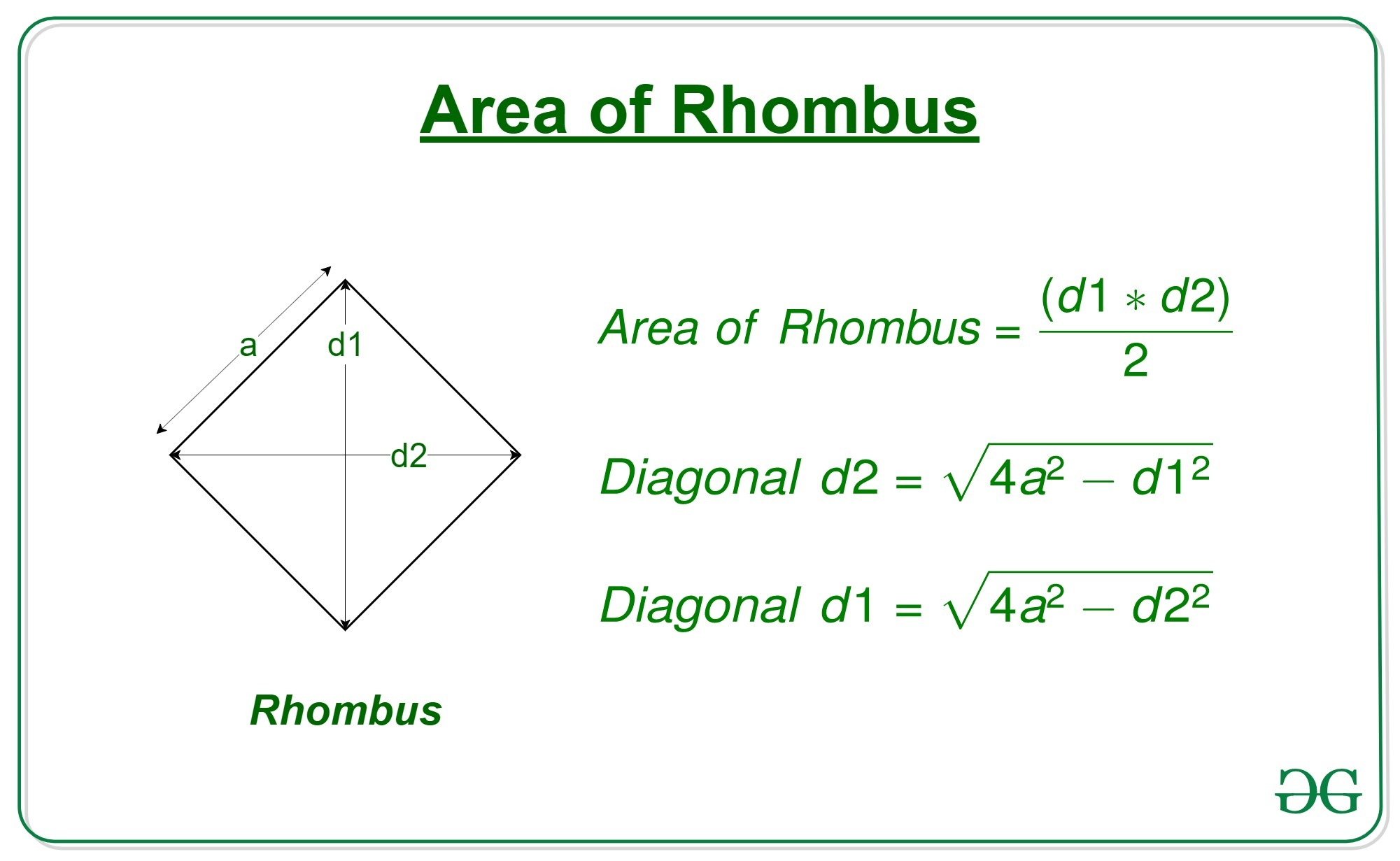# Program to calculate area of a rhombus whose one side and diagonal are given

Given the length of diagonal ‘d1’ of a rhombus and a side ‘a’, the task is to find the area of that rhombus.

A rhombus is a polygon having 4 equal sides in which both the opposite sides are parallel, and opposite angles are equal.Examples:

```Input: d = 15, a = 10
Output: 99.21567416492215

Input: d = 20, a = 18
Output: 299.3325909419153
```

Approach:

## C++

 `// C++ program to calculate the area of a rhombus ` `// whose one side and one diagonal is given ` `#include ` `using` `namespace` `std; ` ` `  `// function to calculate the area of the rhombus ` `double` `area(``double` `d1, ``double` `a) ` `{ ` `     `  `    ``// Second diagonal ` `    ``double` `d2 = ``sqrt``(4 * (a * a) - d1 * d1); ` ` `  `    ``// area of rhombus ` `    ``double` `area = 0.5 * d1 * d2; ` ` `  `    ``// return the area ` `    ``return` `area; ` `} ` ` `  `// Driver code ` `int` `main() ` `{ ` `    ``double` `d = 7.07; ` `    ``double` `a = 5; ` `    ``printf``(``"%0.8f"``, area(d, a)); ` `} ` ` `  `// This code is contributed by Mohit Kumar `

## Java

 `// Java program to calculate the area of a rhombus ` `// whose one side and one diagonal is given ` `class` `GFG ` `{ ` ` `  `    ``// function to calculate the area of the rhombus ` `    ``static` `double` `area(``double` `d1, ``double` `a) ` `    ``{ ` `         `  `        ``// Second diagonal ` `        ``double` `d2 = Math.sqrt(``4` `* (a * a) - d1 * d1); ` `     `  `        ``// area of rhombus ` `        ``double` `area = ``0.5` `* d1 * d2; ` `     `  `        ``// return the area ` `        ``return` `area; ` `    ``} ` `     `  `    ``// Driver code ` `    ``public` `static` `void` `main (String[] args)  ` `    ``{ ` `        ``double` `d = ``7.07``; ` `        ``double` `a = ``5``; ` `        ``System.out.println(area(d, a)); ` `    ``} ` `} ` ` `  `// This code is contributed by AnkitRai01 `

## Python3

 `# Python program to calculate ` `# the area of a rhombus ` `# whose one side and  ` `# one diagonal is given ` ` `  `# function to calculate  ` `# the area of the rhombus ` `def` `area(d1, a): ` `     `  `    ``# Second diagonal ` `    ``d2 ``=` `(``4``*``(a``*``*``2``) ``-` `d1``*``*``2``)``*``*``0.5` `     `  `    ``# area of rhombus ` `    ``area ``=` `0.5` `*` `d1 ``*` `d2 ` `     `  `    ``# return the area ` `    ``return``(area) ` ` `  `# driver code ` `d ``=` `7.07` `a ``=` `5` `print``(area(d, a)) `

## C#

 `// C# program to calculate the area of a rhombus ` `// whose one side and one diagonal is given ` `using` `System; ` ` `  `class` `GFG ` `{ ` ` `  `    ``// function to calculate the area of the rhombus ` `    ``static` `double` `area(``double` `d1, ``double` `a) ` `    ``{ ` `         `  `        ``// Second diagonal ` `        ``double` `d2 = Math.Sqrt(4 * (a * a) - d1 * d1); ` `     `  `        ``// area of rhombus ` `        ``double` `area = 0.5 * d1 * d2; ` `     `  `        ``// return the area ` `        ``return` `area; ` `    ``} ` `     `  `    ``// Driver code ` `    ``public` `static` `void` `Main (String []args)  ` `    ``{ ` `        ``double` `d = 7.07; ` `        ``double` `a = 5; ` `        ``Console.WriteLine(area(d, a)); ` `    ``} ` `} ` ` `  `// This code is contributed by Arnab Kundu `

Output:

```24.999998859949972
```

GeeksforGeeks has prepared a complete interview preparation course with premium videos, theory, practice problems, TA support and many more features. Please refer Placement 100 for details

My Personal Notes arrow_drop_upIf you like GeeksforGeeks and would like to contribute, you can also write an article using contribute.geeksforgeeks.org or mail your article to contribute@geeksforgeeks.org. See your article appearing on the GeeksforGeeks main page and help other Geeks.

Please Improve this article if you find anything incorrect by clicking on the "Improve Article" button below.